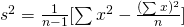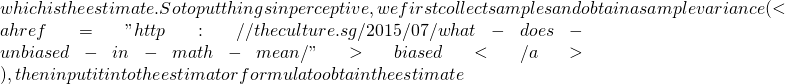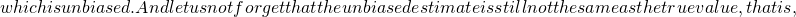What is a good estimate?Some of my student asked me how good is an estimate and how do we measure it up.

Let us first consider the difference between an estimate and estimator. We are all familiar with the following formulaThe above formula is an estimator. It allows us to input our collected data and values to %latex s^{2}latex s^{2}latex s^{2} \ne {\sigma}^{2}\$

So there are definitely more factors that attributes to a good estimate, unbiased is one. The next in line will be consistent. We question if the estimate is consistent, this means to consider if the estimate tends to the true value as n tends to infinity.Not readable? Change text.